20 Options
Many functions in the Wolfram Language have options that determine the details of how they work. For example, in making a plot, you can use PlotTheme"Web" to use a web-oriented visual theme. On a keyboard, the is automatically formed if you type -> (i.e. - followed by >).
 In:=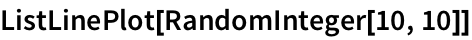Out=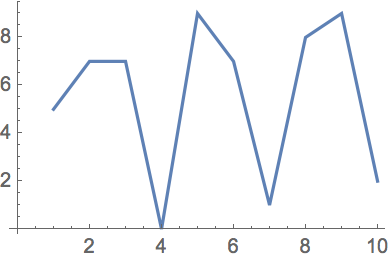A plot with the PlotTheme option given as "Web":
 In:=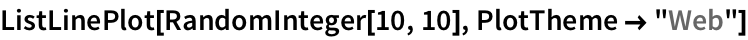Out=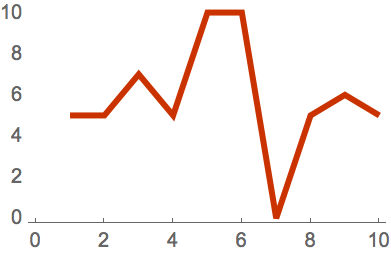A plot with the PlotTheme option given as "Detailed":
 In:=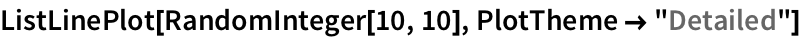Out=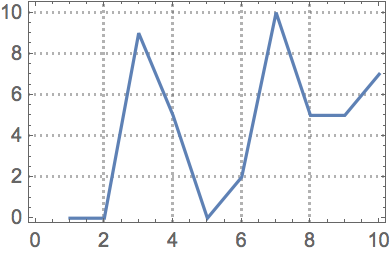A plot with the PlotTheme option given as "Marketing":
 In:=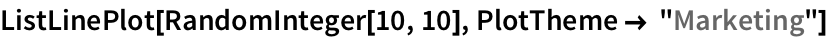Out=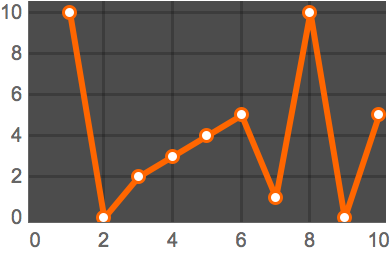You can add more options. For example, Filling specifies what filling to add to a plot.
Fill the plot to the axis:
 In:=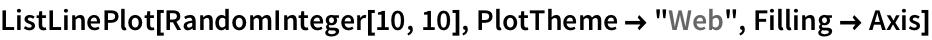Out=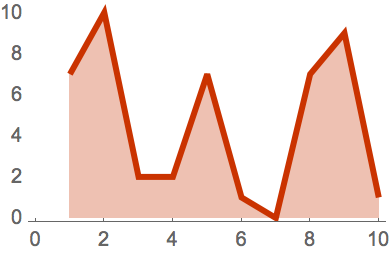Background lets you specify a background color.
 In:=Out=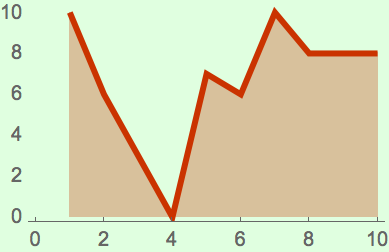One option thats often useful for graphics is PlotRange, which specifies what range of values to include in a plot. With the default , the system will try to automatically show the interesting part of the plot. shows all values.
 In:=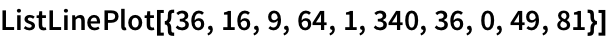Out=PlotRangeAll says to include all points:
 In:=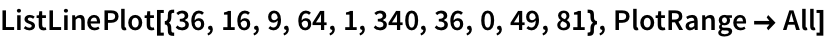Out=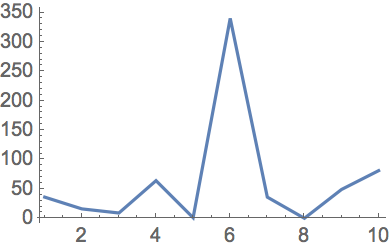specifies to show values up to 30:
 In:=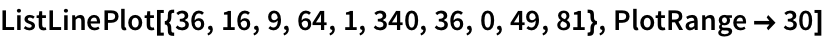Out=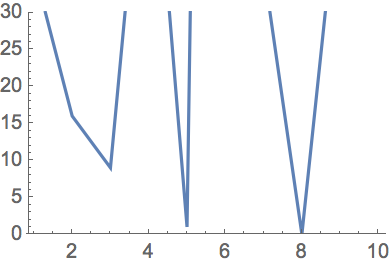PlotRange{20, 100} specifies to show values between 20 and 100:
 In:=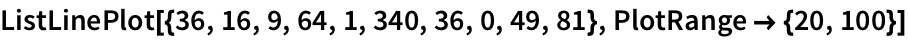Out=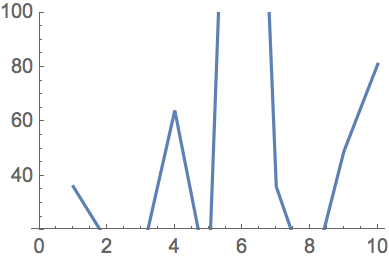You can specify ranges for all types of graphics. In GeoListPlot and GeoGraphics you can use the option GeoRange to specify what part of the world to include in a plot.
 In:=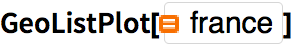Out=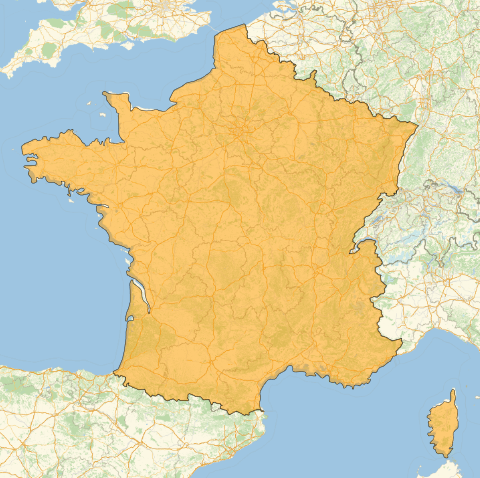In:=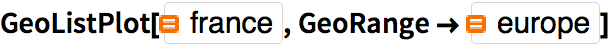Out=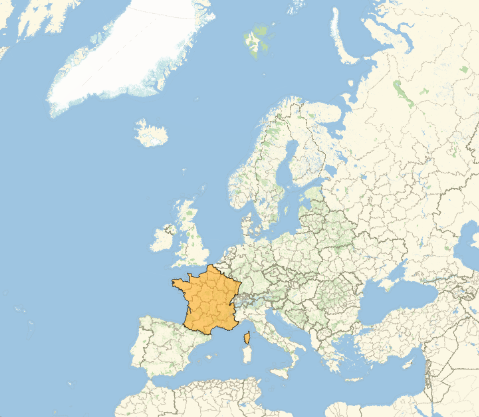GeoRangeAll specifies to use the whole world:
 In:=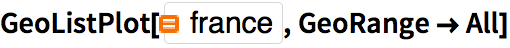Out=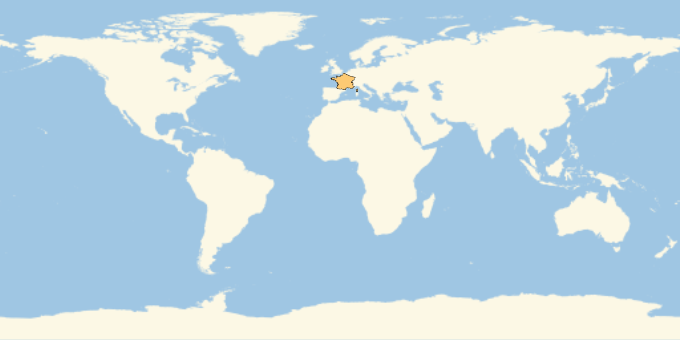There are many other options for GeoListPlot. For example GeoBackground specifies what kind of background should be used. GeoLabels adds labels. Joined makes the points be joined.
 In:=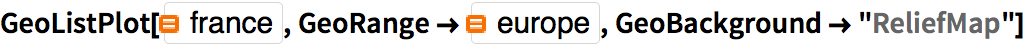Out=In:=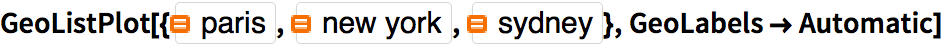Out=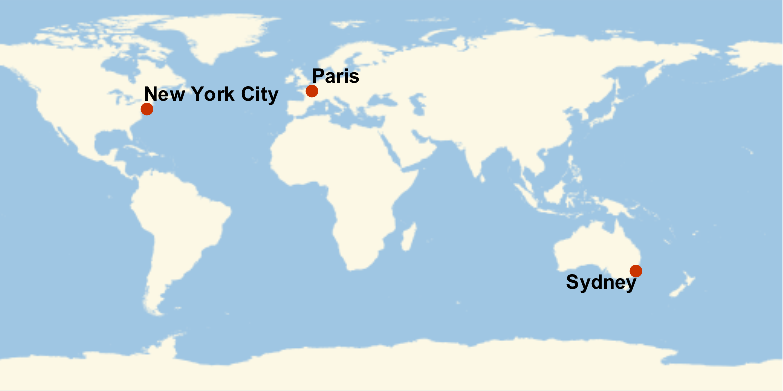Say its True that the points should be joined:
 In:=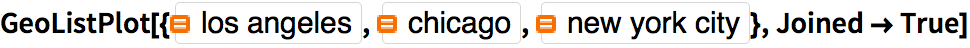Out=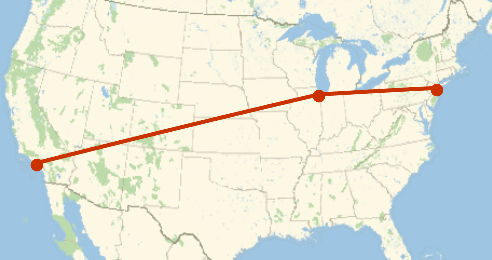The function ListLinePlot has 57 different options you can set; GeoListPlot has 54. Some options are common to all graphics functions. For example, AspectRatio determines the overall shape of graphics, specifying the ratio of height to width.
With an aspect ratio of 1/3, the plot is 3 times wider than it is tall:
 In:=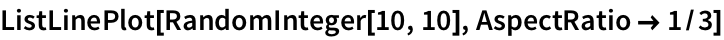Out=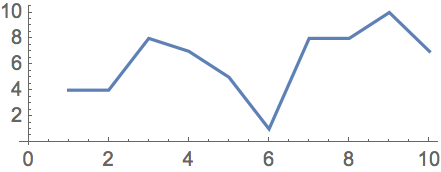The option ImageSize specifies the overall size of graphics.
 In:=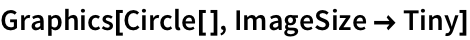Out=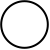Draw circles with specific image sizes between 5 and 50 pixels:
 In:=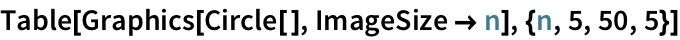Out=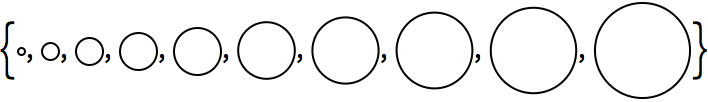Its not just Graphics that allows options. Lots of other functions do too. An example is Style, which supports many options.
 In:=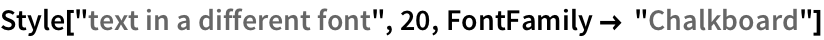Out=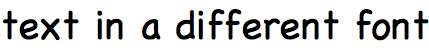In:=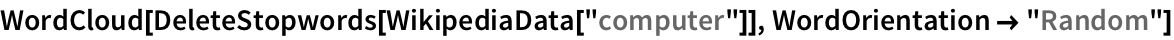Out=Grid has many options. The Frame option controls whether and how a frame is drawn.
Create a multiplication table with a frame around each entry:
 In:=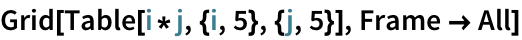Out=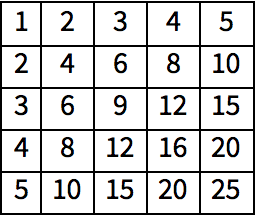In:=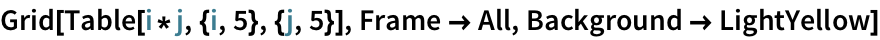Out=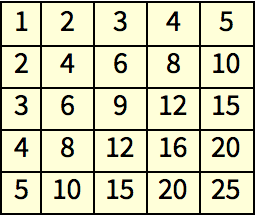PlotTheme theme for a plot (e.g. "Web", "Detailed", etc.) Filling filling to add to a plot (Axis, Bottom, etc.) PlotRange range of values to include in a plot (All, etc.) GeoRange geo range to include (All, specific country, etc.) GeoBackground background map ("ReliefMap", "OutlineMap", etc.) GeoLabels labels to add to a map (e.g. Automatic) Joined whether to make points be joined (True, False) Background background color AspectRatio ratio of height to width ImageSize size in pixels Frame whether to include a frame (True, All, etc.) FontFamily family of font to use (e.g. "Helvetica") WordOrientation how to orient words in a word cloud
20.1Create a list plot of Range themed for the web. »
Expected output:
 Out[]=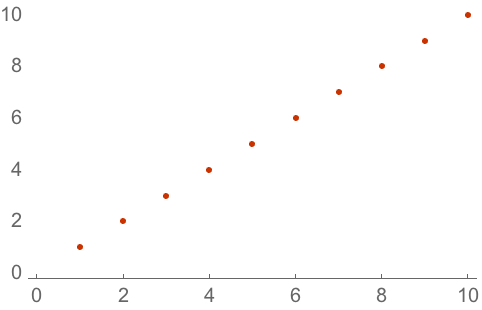Answer & check your solution
20.2Create a list plot of Range with filling to the axis. »
Expected output:
 Out[]=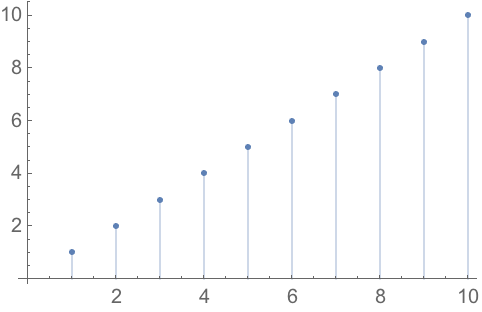Answer & check your solution
20.3Create a list plot of Range with a yellow background. »
Expected output:
 Out[]=Answer & check your solution
20.4Create a map of the world with Australia highlighted. »
Expected output:
 Out[]=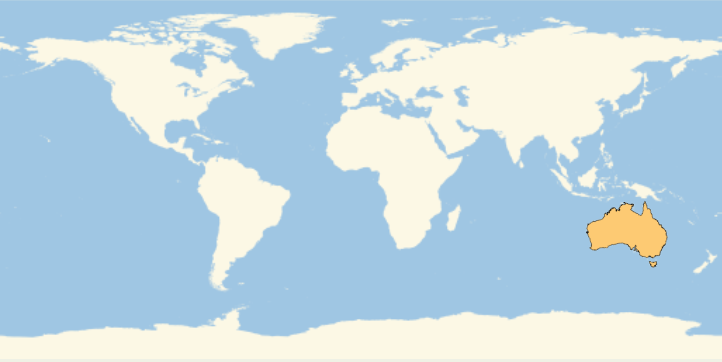Answer & check your solution
20.5Create a map of the Indian Ocean with Madagascar highlighted. »
Expected output:
 Out[]=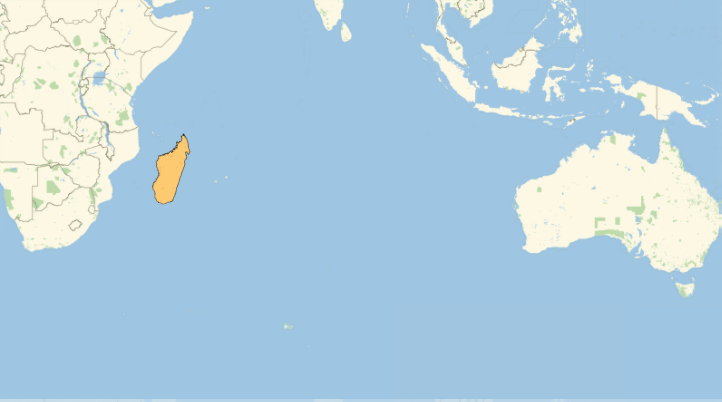Answer & check your solution
20.6Use GeoGraphics to create a map of South America showing topography (relief map). »
Expected output:
 Out[]=Answer & check your solution
20.7Make a map of Europe with France, Finland and Greece highlighted and labeled. »
Expected output:
 Out[]=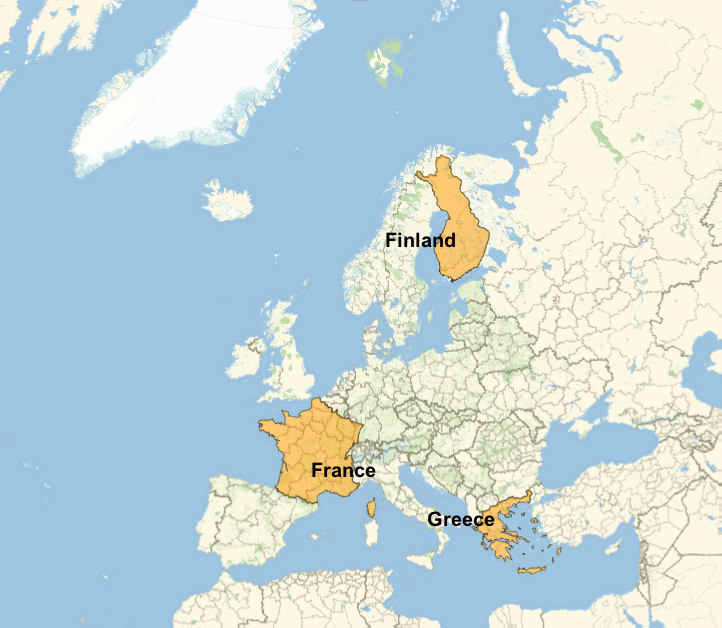Answer & check your solution
20.8Make a 12×12 multiplication table as a grid with white type on a black background. »
Expected output:
 Out[]=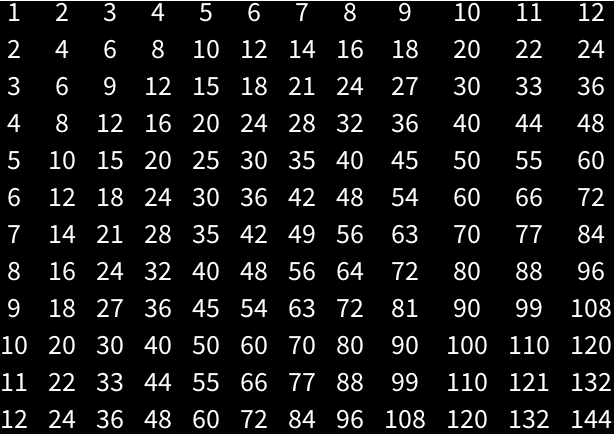Answer & check your solution
20.9Make a list of 100 disks with random integer image sizes up to 40. »
Sample expected output:
 Out[]=Answer & check your solution
20.10Make a list of pictures of regular pentagons with image size 30 and aspect ratios from 1 to 10. »
Expected output:
 Out[]=Answer & check your solution
20.11Make a Manipulate that varies the size of a circle between 5 and 500. »
Sample expected output:
 Out[]=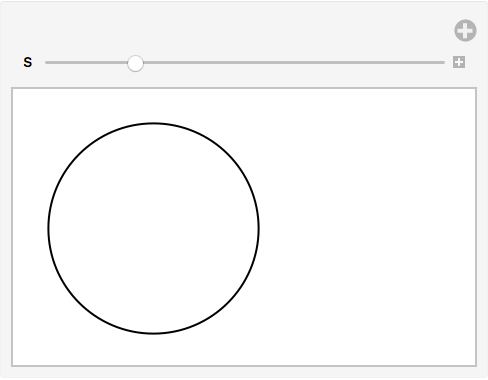Answer & check your solution
20.12Create a framed 10×10 grid of random colors. »
Sample expected output:
 Out[]=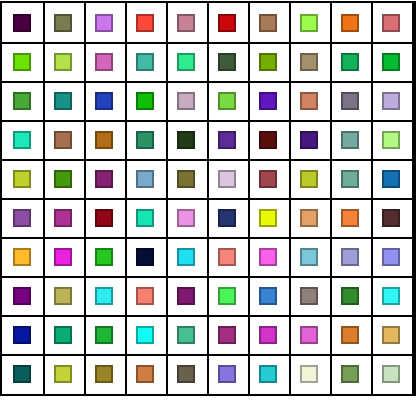Answer & check your solution
Expected output:
 Out[]=Answer & check your solution
+20.1Create a list plot of Range with a frame included. »
Expected output:
 Out[]=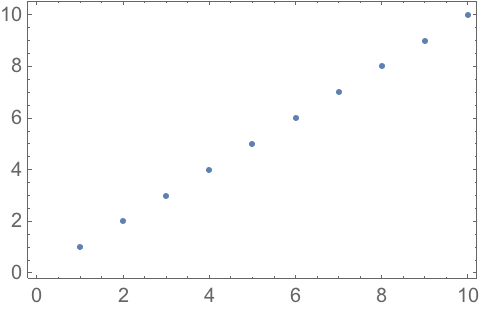Answer & check your solution
+20.2Create a list plot of Range with a yellow background and a frame. »
Expected output:
 Out[]=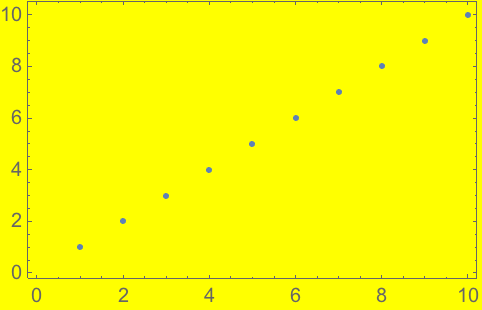Answer & check your solution
+20.3Make a list of list plots of Range, with sizes ranging from 50 to 150 in steps of 10. »
Expected output:
 Out[]=Answer & check your solution
+20.4Make a line plot of the first 20 squares, filling to the axis. »
Expected output:
 Out[]=Answer & check your solution
+20.5Create a relief map of the region around Mount Everest, drawing a disk of radius 100 miles.  »
Expected output:
 Out[]=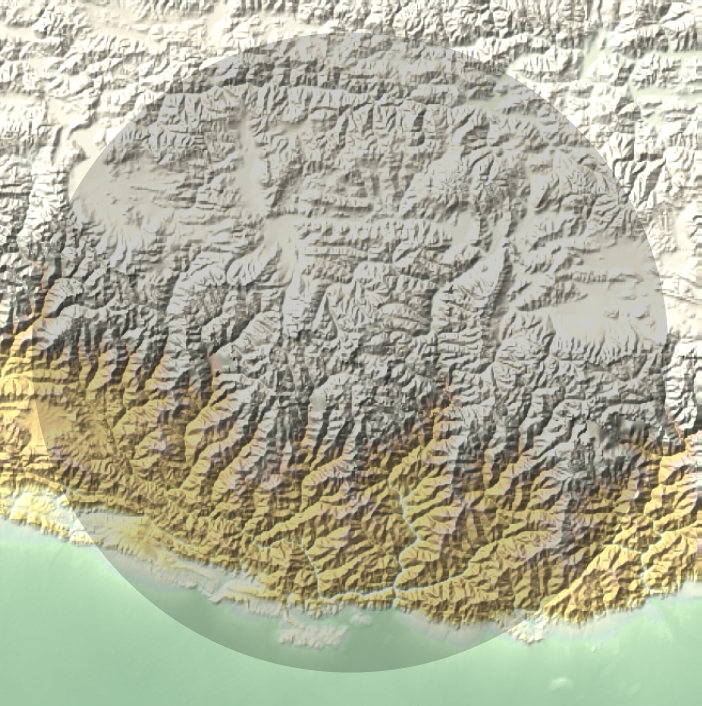Answer & check your solution
+20.6Create a list plot of Range with an aspect ratio of 1. »
Expected output:
 Out[]=Answer & check your solution
+20.7Make a Manipulate that varies the aspect ratio of a picture of a regular hexagon from 0.1 to 5. »
Expected output:
 Out[]=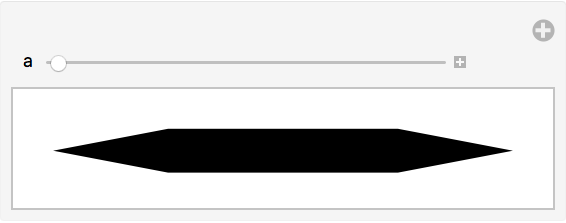Answer & check your solution
+20.8Make a plot of the air temperature in Paris over the past week with the plot range restricted between 50 and 80 degrees. »
Sample expected output:
 Out[]=Answer & check your solution
How can I get a list of the options for a function?
Look at the documentation. Or use for example . Also, whenever you start typing the name of an option, youll see a menu of possible completions.
How do I find out the possible settings for an option?
Look at the documentation for that option. Also, when you type , youll typically get a menu of possible common settings.
What is optvalue internally?
Its Rule[opt, value]. Rules are used in lots of places in the Wolfram Language. ab is usually read aloud as a goes to b or a arrow b.
When are values of options given as strings?
Only a small set of standard option settings (such as Automatic, None and All) are not strings. Specialized settings for particular options are normally strings.
Can one reset the default for an option?
Yes, using SetOptions. Though you have to be careful not to forget that youve done it.